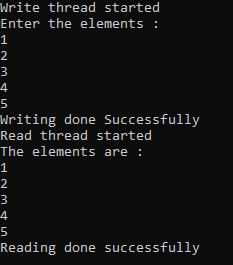• Last Updated : 18 May, 2021

An array is a collection of items stored at contiguous memory locations. The idea is to store multiple items of the same type together. This makes it easier to calculate the position of each element by simply adding an offset to a base value, i.e., the memory location of the first element of the array (generally denoted by the name of the array). Arrays are used to store homogeneous data. The conventional way of storing the elements in an array and reading the elements from an array takes a lot of time if there are numerous elements.Attention reader! Don’t stop learning now. Get hold of all the important Java Foundation and Collections concepts with the Fundamentals of Java and Java Collections Course at a student-friendly price and become industry ready. To complete your preparation from learning a language to DS Algo and many more,  please refer Complete Interview Preparation Course.

Writing and reading elements in an array is a small problem where each element is first added to the array and then the entire array is read element by element and printed on the console. But when the number of elements is too large, it could take a lot of time. But this could be solved by dividing the writing and reading tasks into parts.

This could be done by using multi-threading where each core of the processor is used. In this case, two threads are used, where one thread is responsible for writing to the array and the other thread is responsible for reading the array. In this way, the performance of a program can be improved as well as the cores of the processor can be utilized. It is better to use one thread for each core. Although one can create as many threads as required for a better understanding of multi-threading.

Approach: This section states the algorithm that is followed to design a program to writing and read elements of an array using multithreading:

• In the first step, two threads will be created.
• One for writing operation and one for reading operation.
• Here the synchronized keyword is used with the array so that only one thread can access the array at a time.
• First, the write operation will be performed on the array.
• Then, the read operation is performed on the array.

Below is the Java program to implement the above approach-

## Java

 `// Java program for the above approach``import` `java.io.*;``import` `java.util.*;``class` `GFG {``    ``public` `static` `void` `main(String[] args)``    ``{``        ``// Array created for 5 elements``        ``int` `a[] = ``new` `int``[``5``];`` ` `        ``// Thread created for write operation``        ``Thread t1 = ``new` `Thread(``new` `Runnable() {``            ``public` `void` `run()``            ``{``                ``// Here the array is being``                ``// synchronized``                ``synchronized` `(a)``                ``{``                    ``Scanner s = ``new` `Scanner(System.in);``                    ``System.out.println(``                        ``"Enter the elements : "``);`` ` `                    ``for` `(``int` `i = ``0``; i < ``5``; i++) {``                        ``a[i] = s.nextInt();``                    ``}`` ` `                    ``System.out.println(``                        ``"Writing done Successfully"``);``                ``}``            ``}``        ``});`` ` `        ``// Thread created for read operation``        ``Thread t2 = ``new` `Thread(``new` `Runnable() {``            ``public` `void` `run()``            ``{``                ``// Here the array is being``                ``// synchronized``                ``synchronized` `(a)``                ``{``                    ``System.out.println(``                        ``"The elements are : "``);`` ` `                    ``for` `(``int` `i = ``0``; i < ``5``; i++) {``                        ``System.out.println(a[i]);``                    ``}`` ` `                    ``System.out.println(``                        ``"Reading done successfully"``);``                ``}``            ``}``        ``});`` ` `        ``// Write thread is started``        ``t1.start();`` ` `        ``// Read thread is started``        ``t2.start();``    ``}``}`

Output:Explanation: Here, firstly the write thread is started and at that time read thread will not interfere as the array is synchronized. Similarly, during reading, write thread will not interfere.

My Personal Notes arrow_drop_up